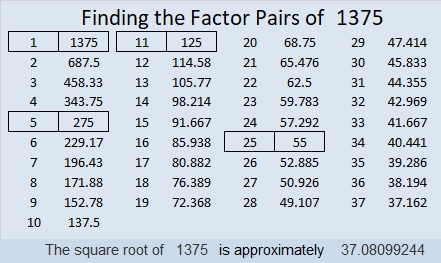# The Cube Root of 1375 is the Smallest. . .

The cube root of 1375 can be simplified. So can the cube roots of 1376 and 1377. There are no smaller consecutive three numbers whose cube roots can make the same claim.Thank you, OEIS.org for alerting me to that very cool fact. It deserves a celebration so I made the graphic above.

Here’s more about the number 1375:

• 1375 is a composite number.
• Prime factorization: 1375 = 5 × 5 × 5 × 11, which can be written 1375 = 5³ × 11
• 1375 has at least one exponent greater than 1 in its prime factorization so √1375 can be simplified. Taking the factor pair from the factor pair table below with the largest square number factor, we get √1375 = (√25)(√55) = 5√55
• The exponents in the prime factorization are 3 and 1. Adding one to each exponent and multiplying we get (3 + 1)(1 + 1) = 4 × 2 = 8. Therefore 1375 has exactly 8 factors.
• The factors of 1375 are outlined with their factor pair partners in the graphic below.1375 is also the hypotenuse of THREE Pythagorean triples:
385-1320-1375 which is (7-24-25) times 55
484-1287-1375 which is 11 times (44-117-125)
825-1100-1375 which is (3-4-5) times 275

# Is There Anything Else Special about the Palindrome 939?

Yes, 939 is a palindrome in base 10, but also all of its factors (1, 3, 313, and 939) are palindromes. It is also palindrome
32223 in BASE 4 because 3(4⁴) + 2(4³) + 2(4²) + 2(4¹) + 3(4⁰) = 939

Okay, that’s nice. Is there anything else special about 939?

The first ten decimal places of the cube root of 939 contain ALL ten digits 0 to 9. That’s unusual, and a reason why 939 is a special number. I made this gif to highlight its uniqueness.make science GIFs like this at MakeaGif

***********

Thank you OEIS.org for informing us of that amazing fact about 939’s cube root.

939 is also the hypotenuse of a Pythagorean triple:
75-936-939 which is 3 times (25-312-313)

• 939 is a composite number.
• Prime factorization: 939 = 3 × 313
• The exponents in the prime factorization are 1 and 1. Adding one to each and multiplying we get (1 + 1)(1 + 1) = 2 × 2 = 4. Therefore 939 has exactly 4 factors.
• Factors of 939: 1, 3, 313, 939
• Factor pairs: 939 = 1 × 939 or 3 × 313
• 939 has no square factors that allow its square root to be simplified. √939 ≈ 30.64310689